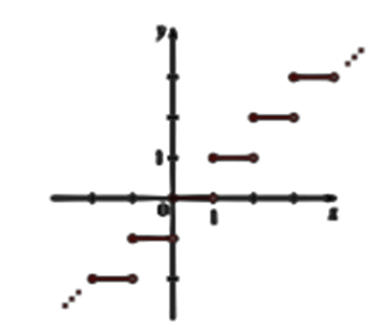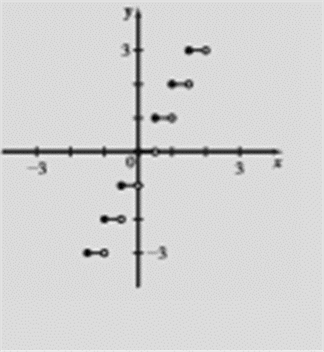# To sketch the graph of f ( x ) = [ | 2 x | ] by using the function graph f ( x ) = [ | x | ] .### Precalculus: Mathematics for Calcu...

6th Edition
Stewart + 5 others
Publisher: Cengage Learning
ISBN: 9780840068071### Precalculus: Mathematics for Calcu...

6th Edition
Stewart + 5 others
Publisher: Cengage Learning
ISBN: 9780840068071

#### Solutions

Chapter 2.5, Problem 67E
To determine

## To sketch the graph of f(x)=[|2x|] by using the function graph f(x)=[|x|] .

Expert Solution

### Explanation of Solution

Given information:

The function is f(x)=[|x|]Graph :

the graph of function f(x)=[|2x|] obtained as:Interpretation: from the above graph it is observed that when f(x)=[|x|] is given, the graph of the function f(x)=[|2x|] is obtained by horizontal stretching the graph of f(x)=[|x|] by the factor of 12 .

### Have a homework question?

Subscribe to bartleby learn! Ask subject matter experts 30 homework questions each month. Plus, you’ll have access to millions of step-by-step textbook answers!